# Kerala Plus Two Maths Previous Question Papers and Answers 2016 In PDF

## Kerala Class 12th Maths Question Paper 2016 Solved – Free Download

Kerala Plus Two (Class 12) maths 2016 question paper with answers are provided here in a downloadable pdf format and also in the text, so that the students can study them offline and obtain them for free. Along with the solutions, maths question paper 2016 Class 12 is also attached here for reference. 12th Students are able to access all the Kerala board previous year maths question papers. The solutions provided by BYJU’S will assist the students for better preparation. Solving 2016 Maths question papers and also the previous year papers for Class 12 will equip the students to learn better time management skills and can face the board exams without any fear.

### KBPE Plus Two (Class 12th) Maths Question Paper With Solutions 2016

#### QUESTION PAPER CODE 1018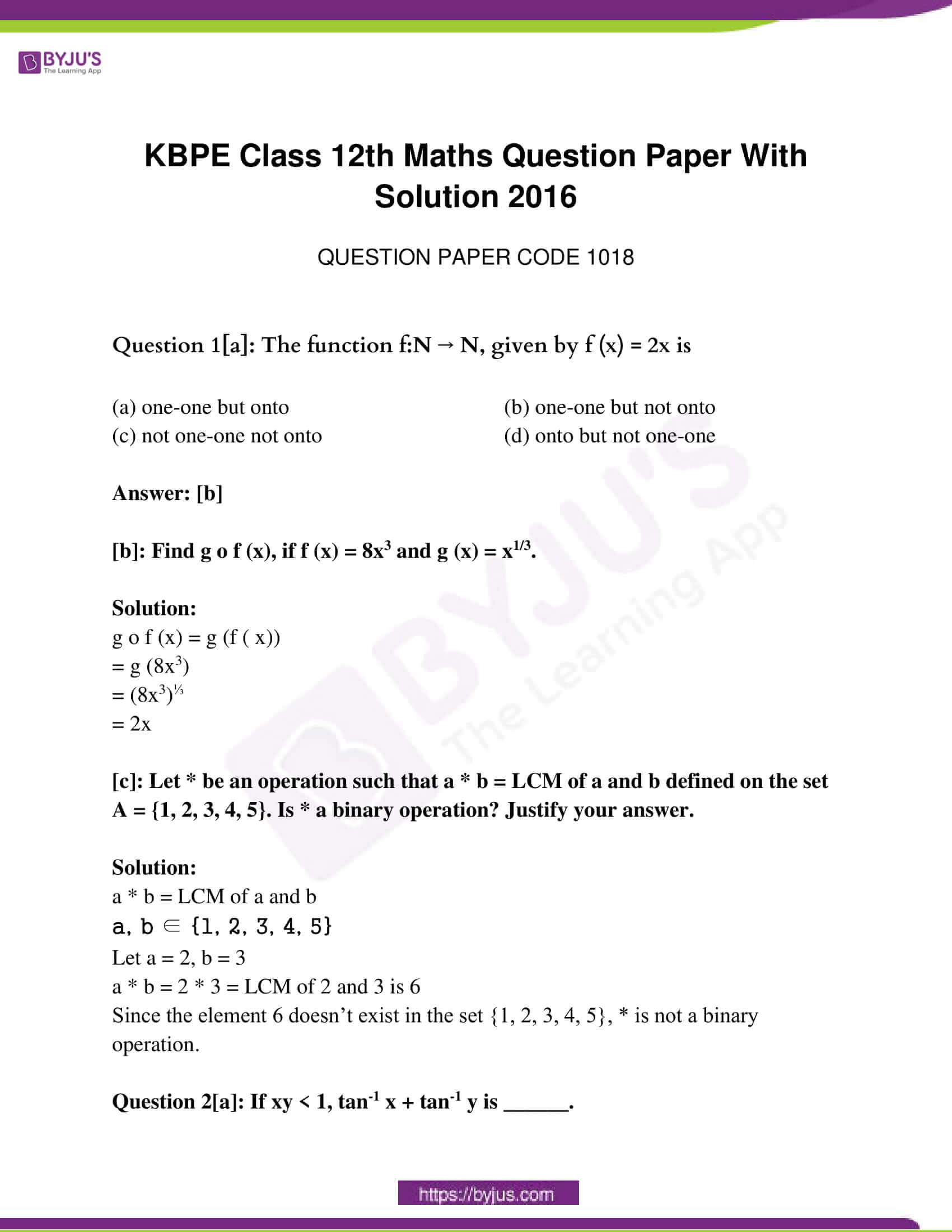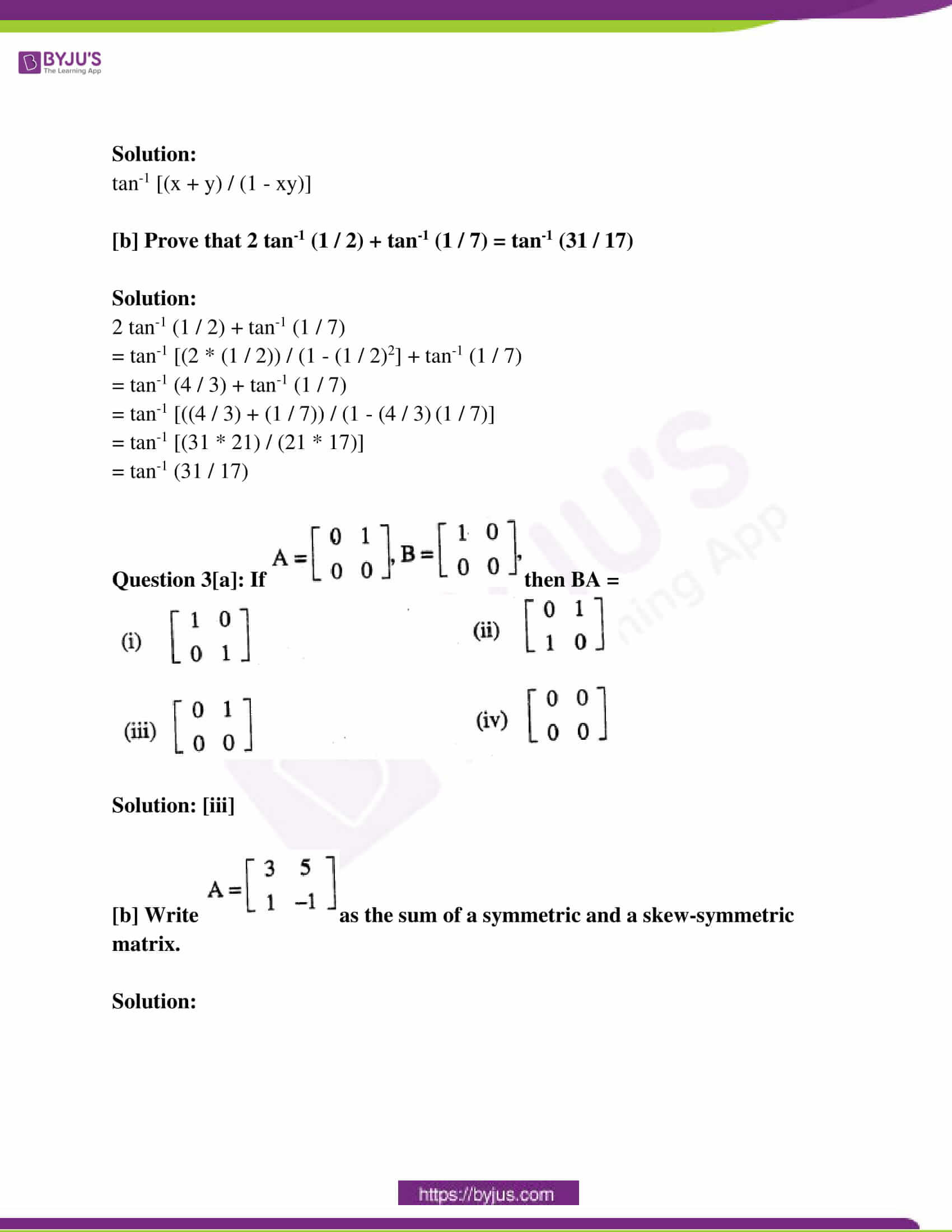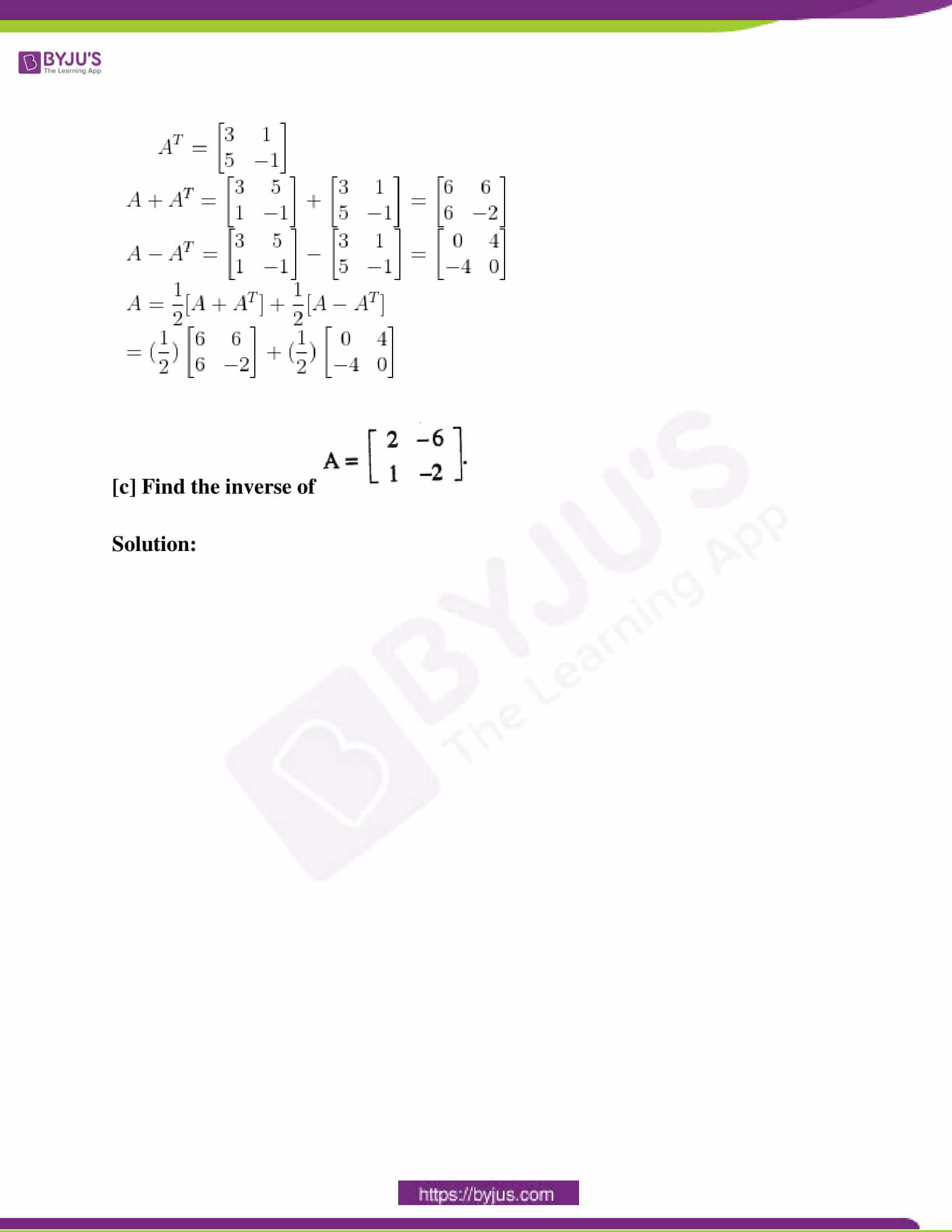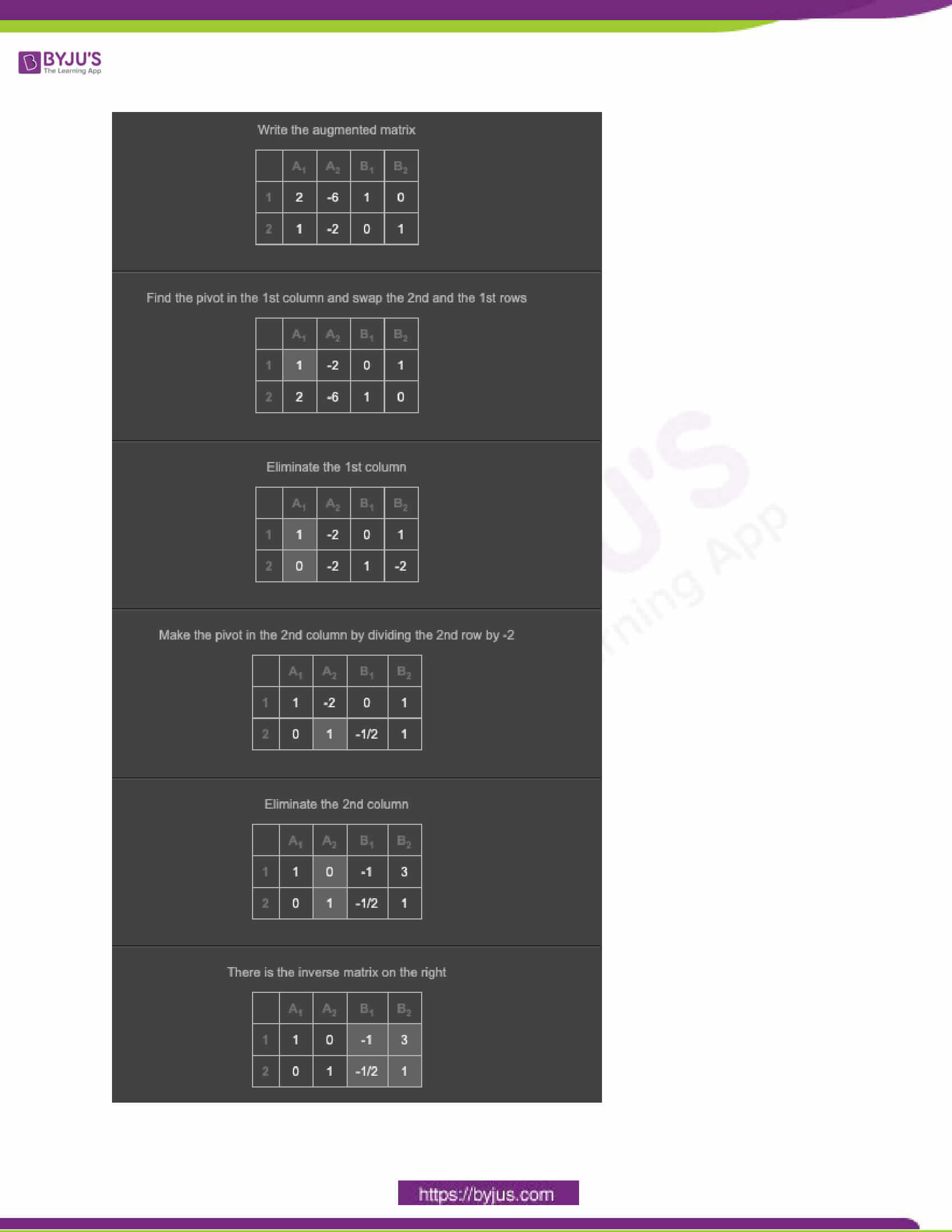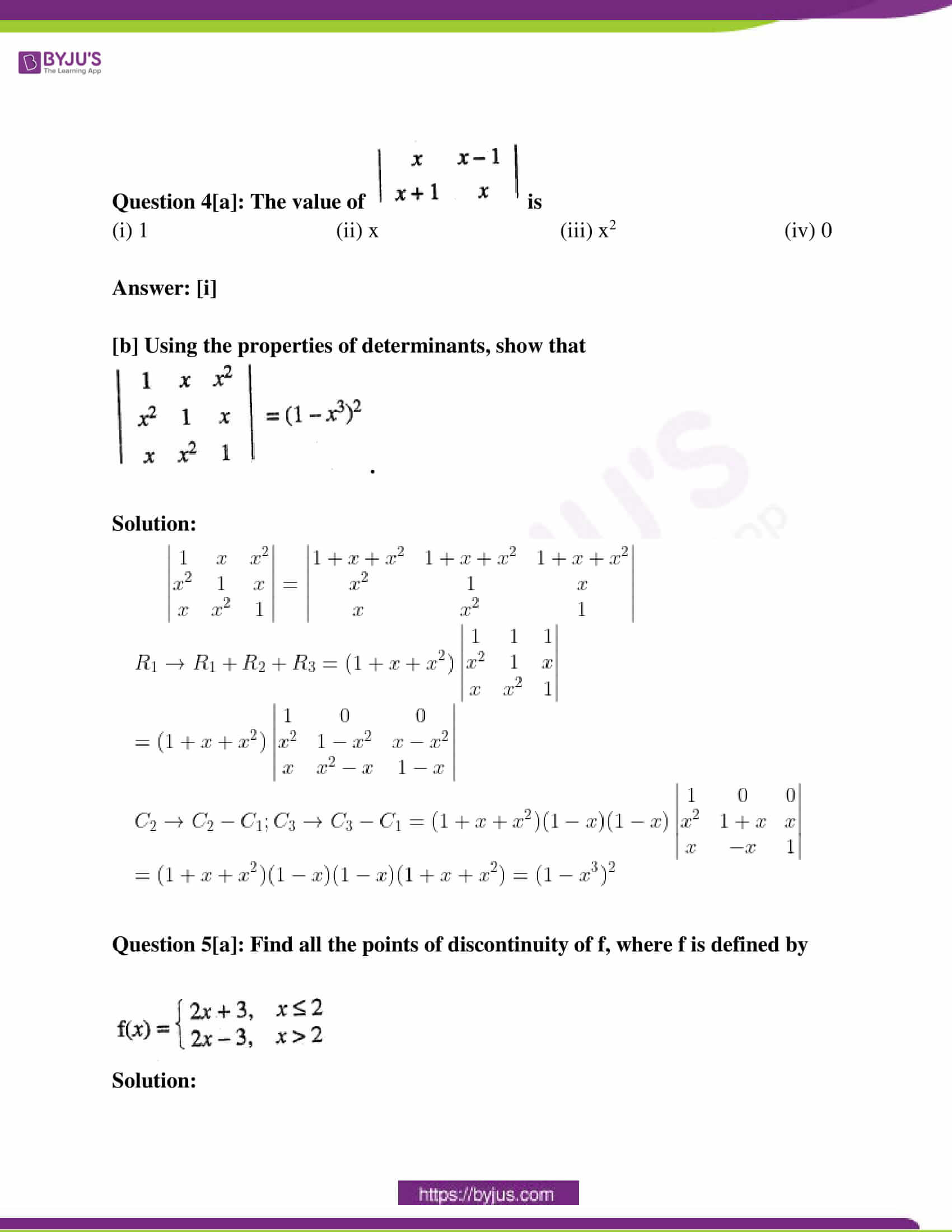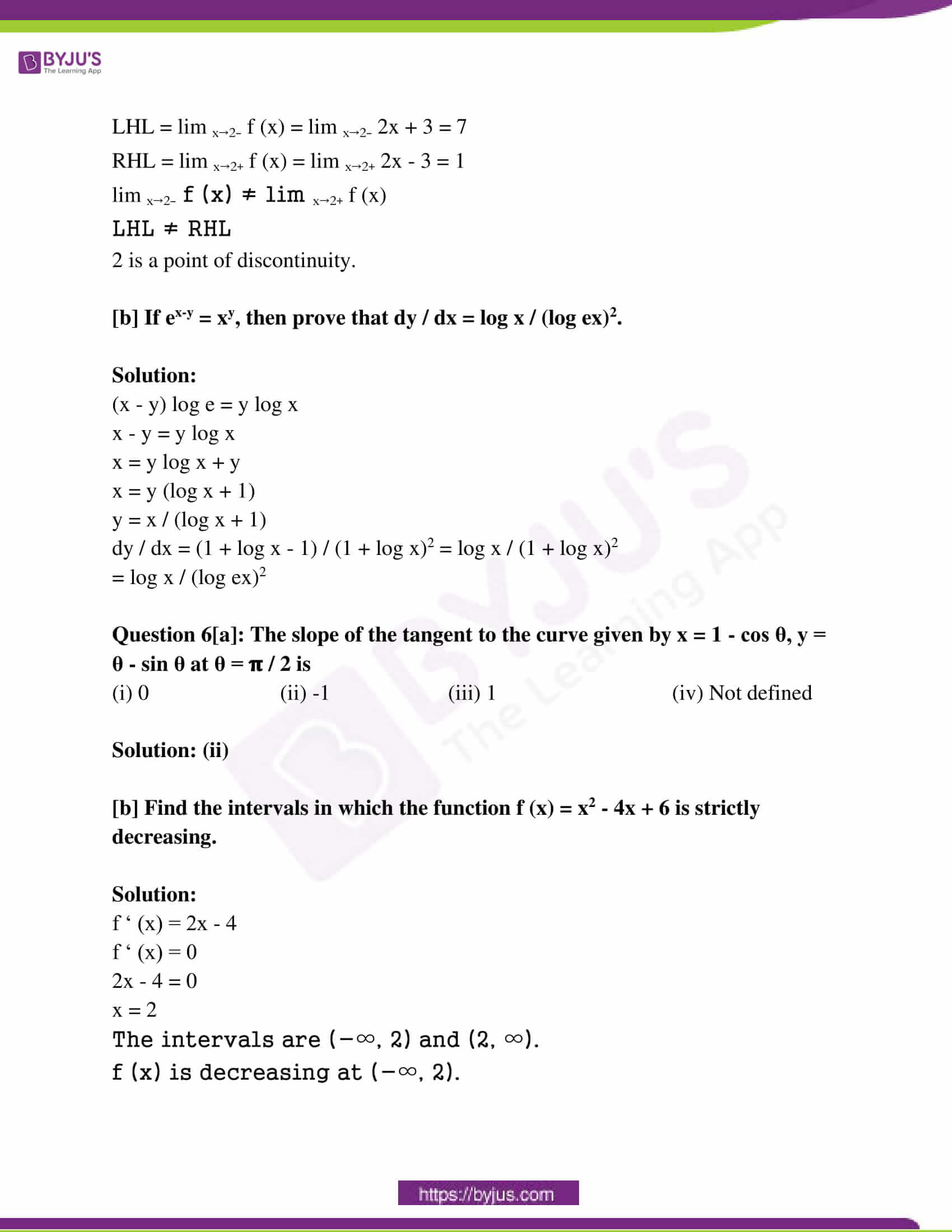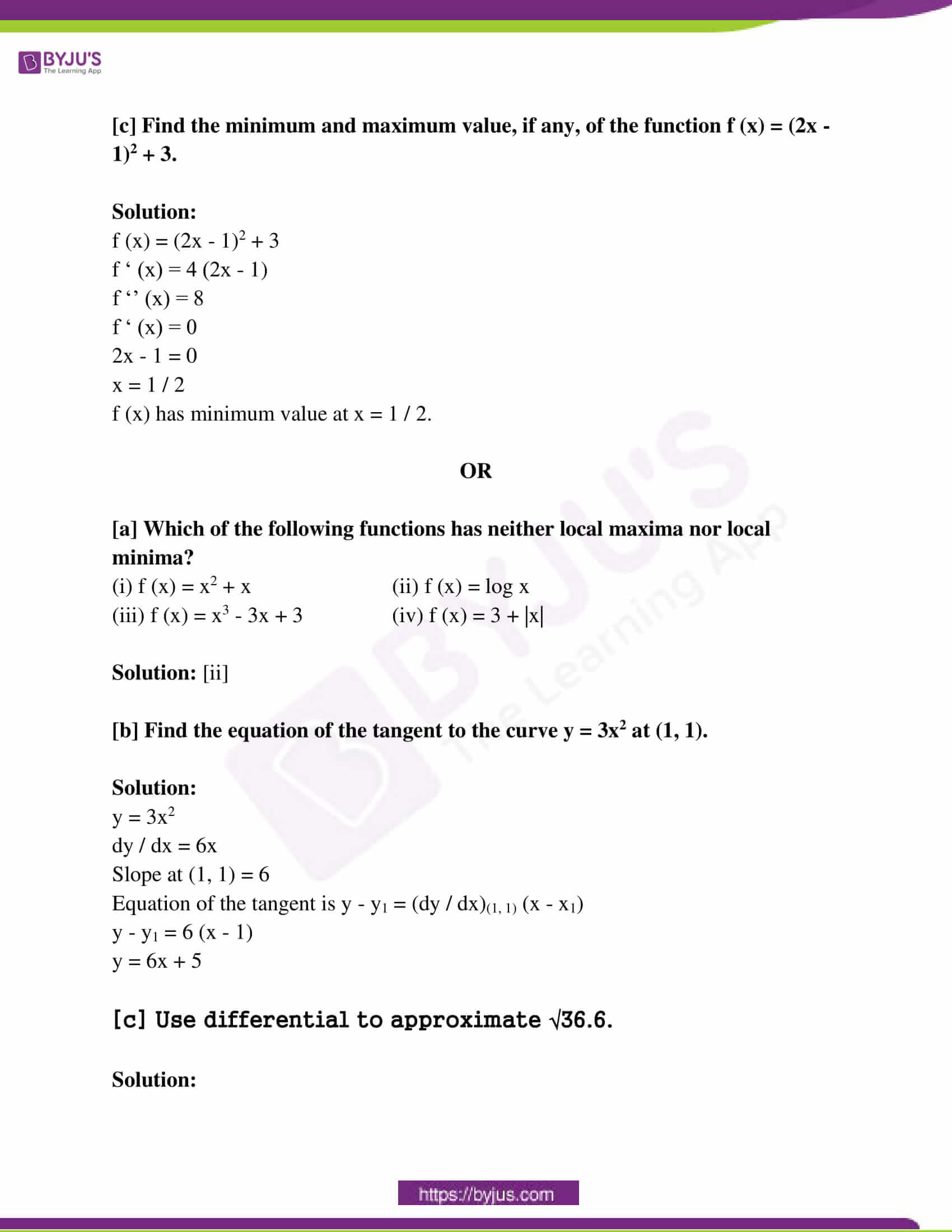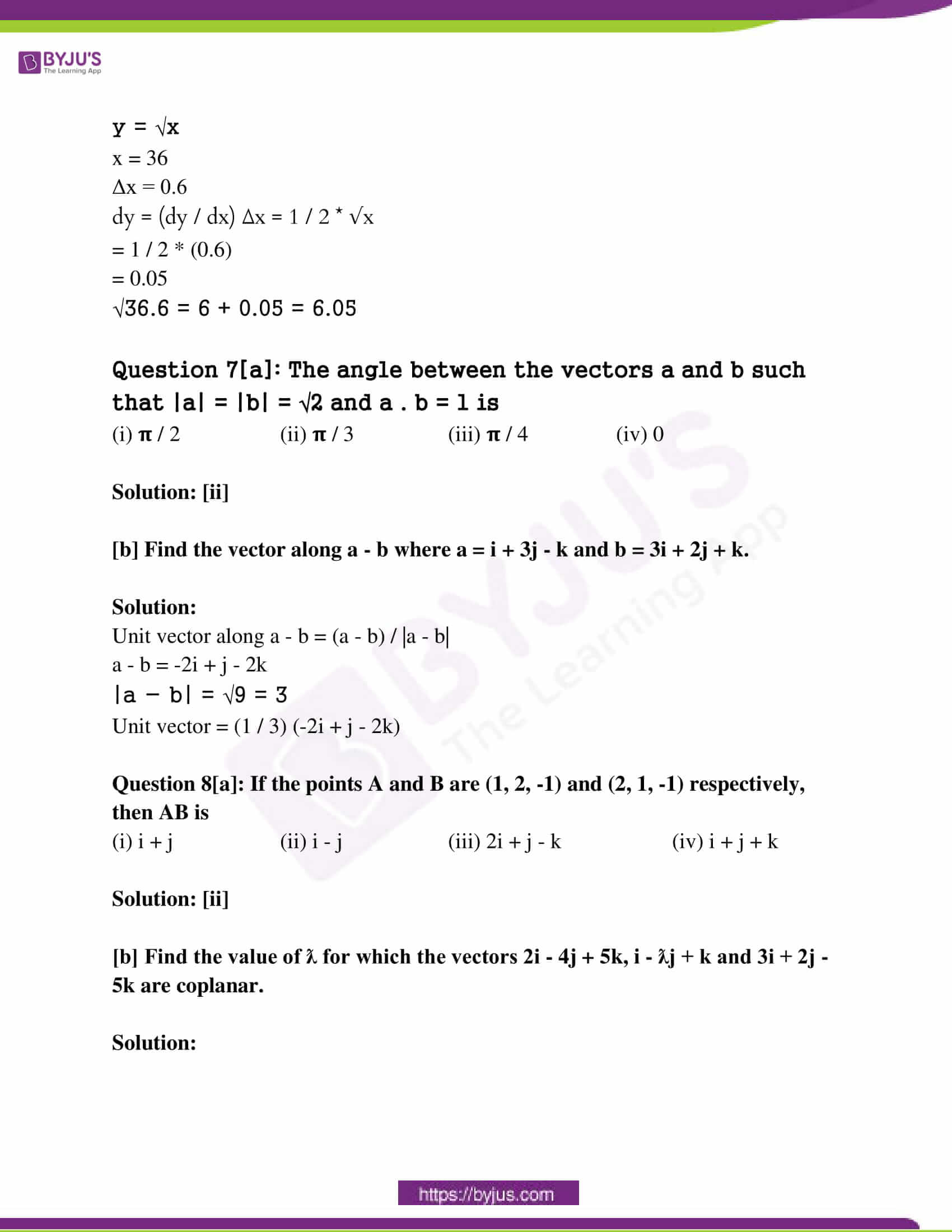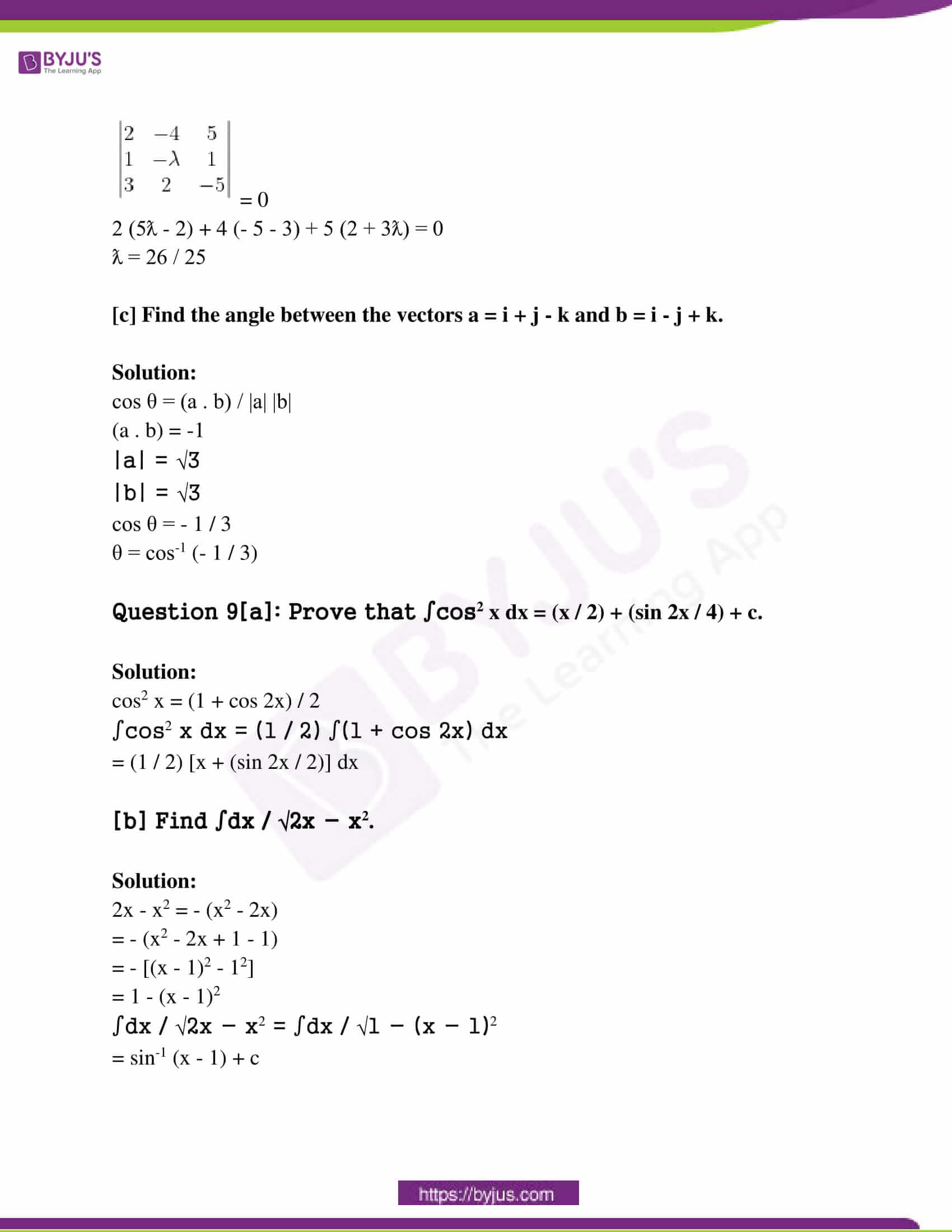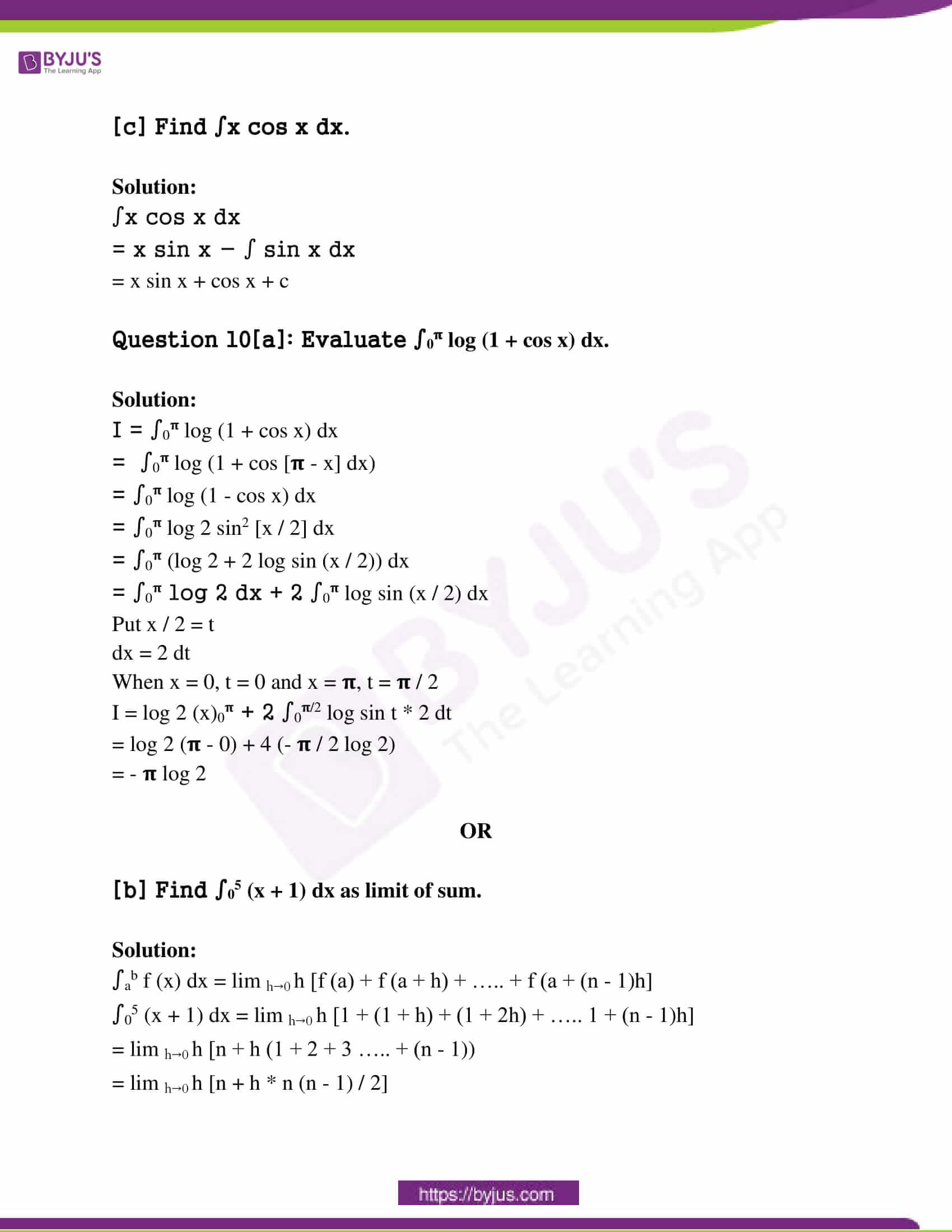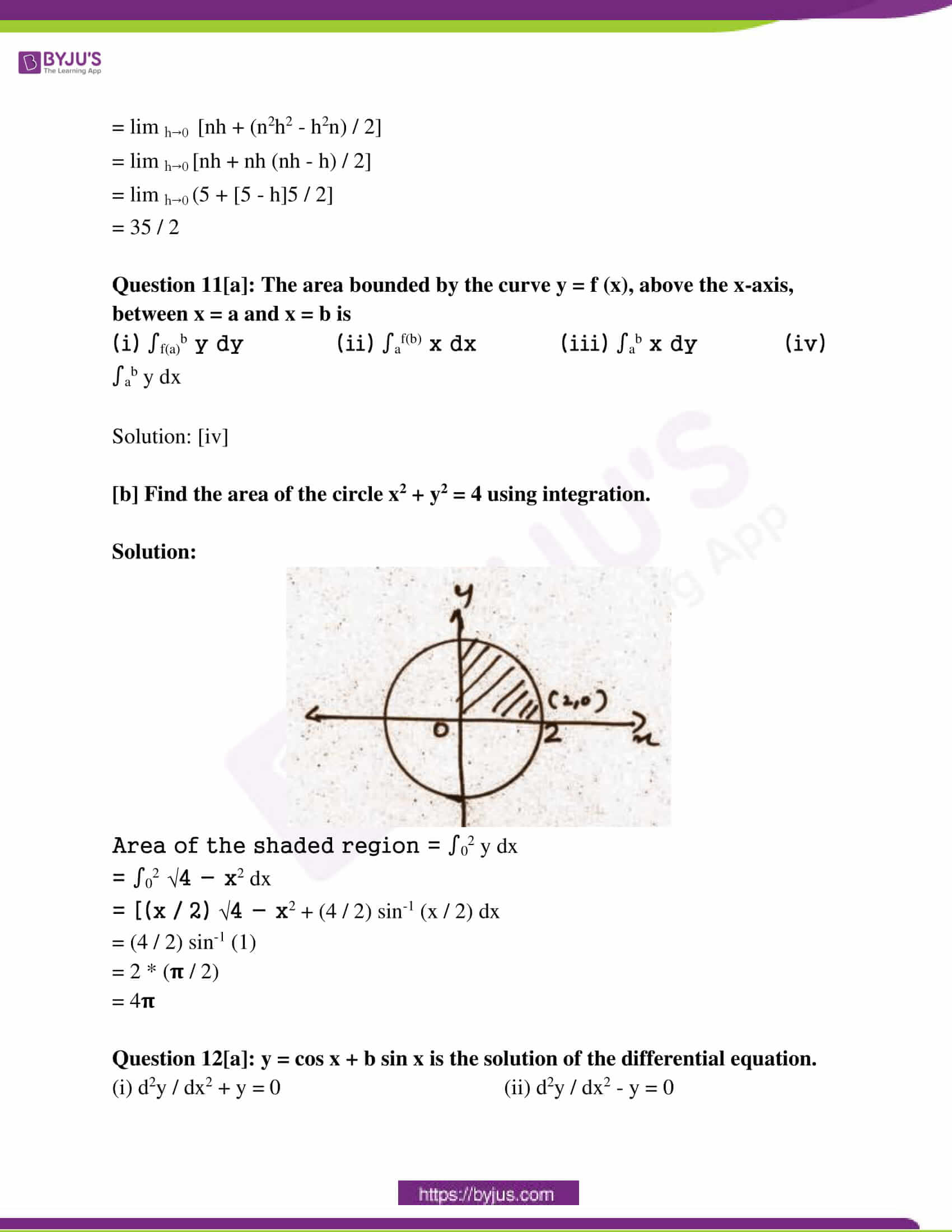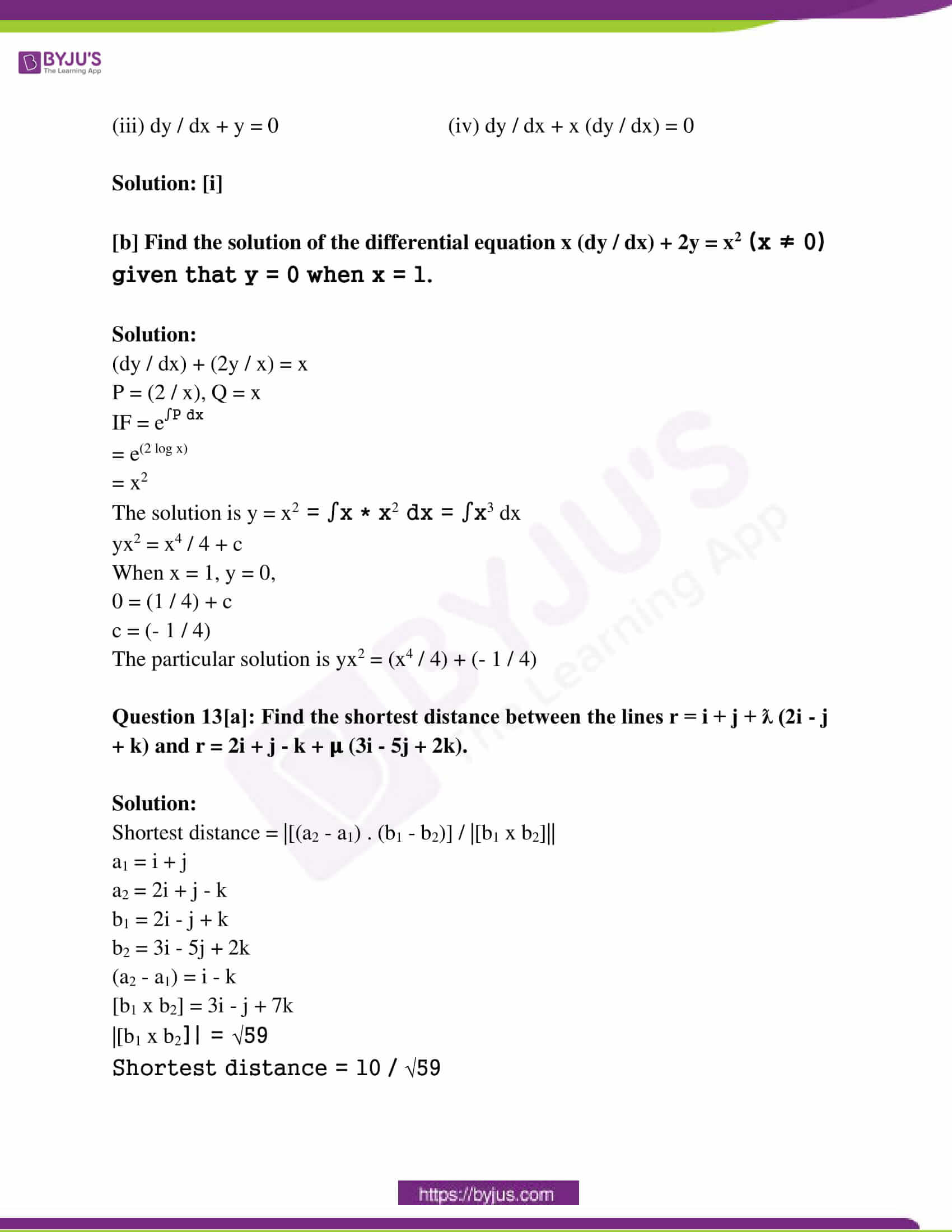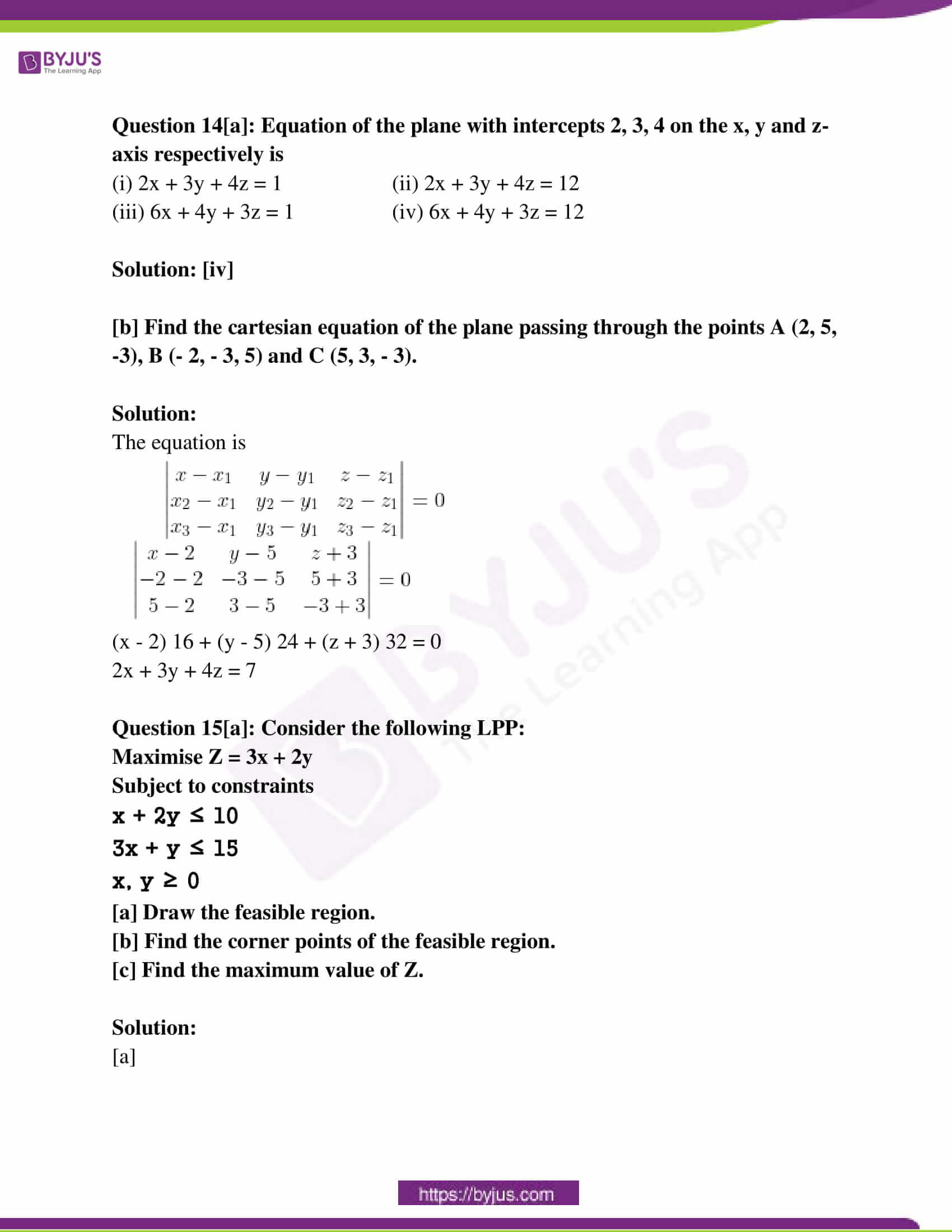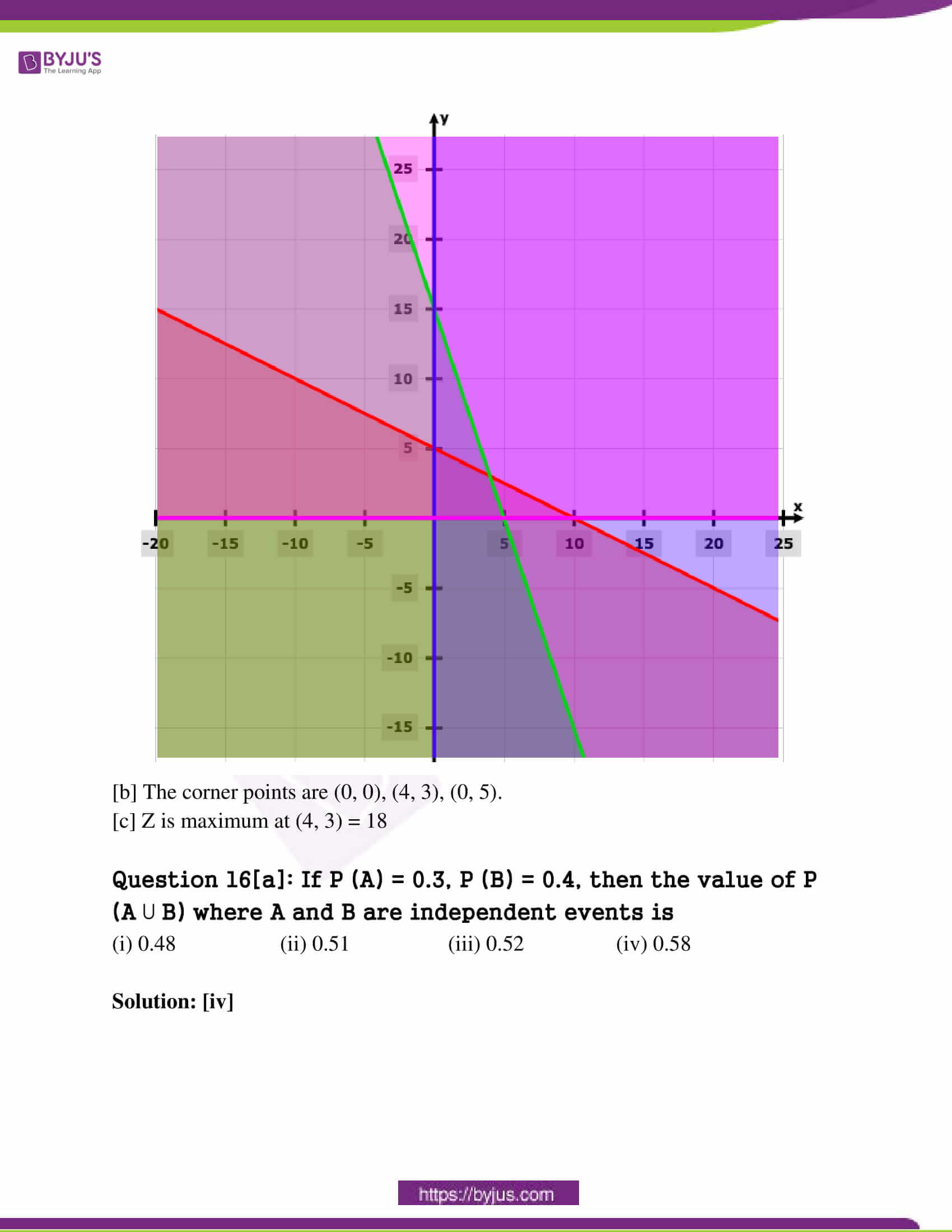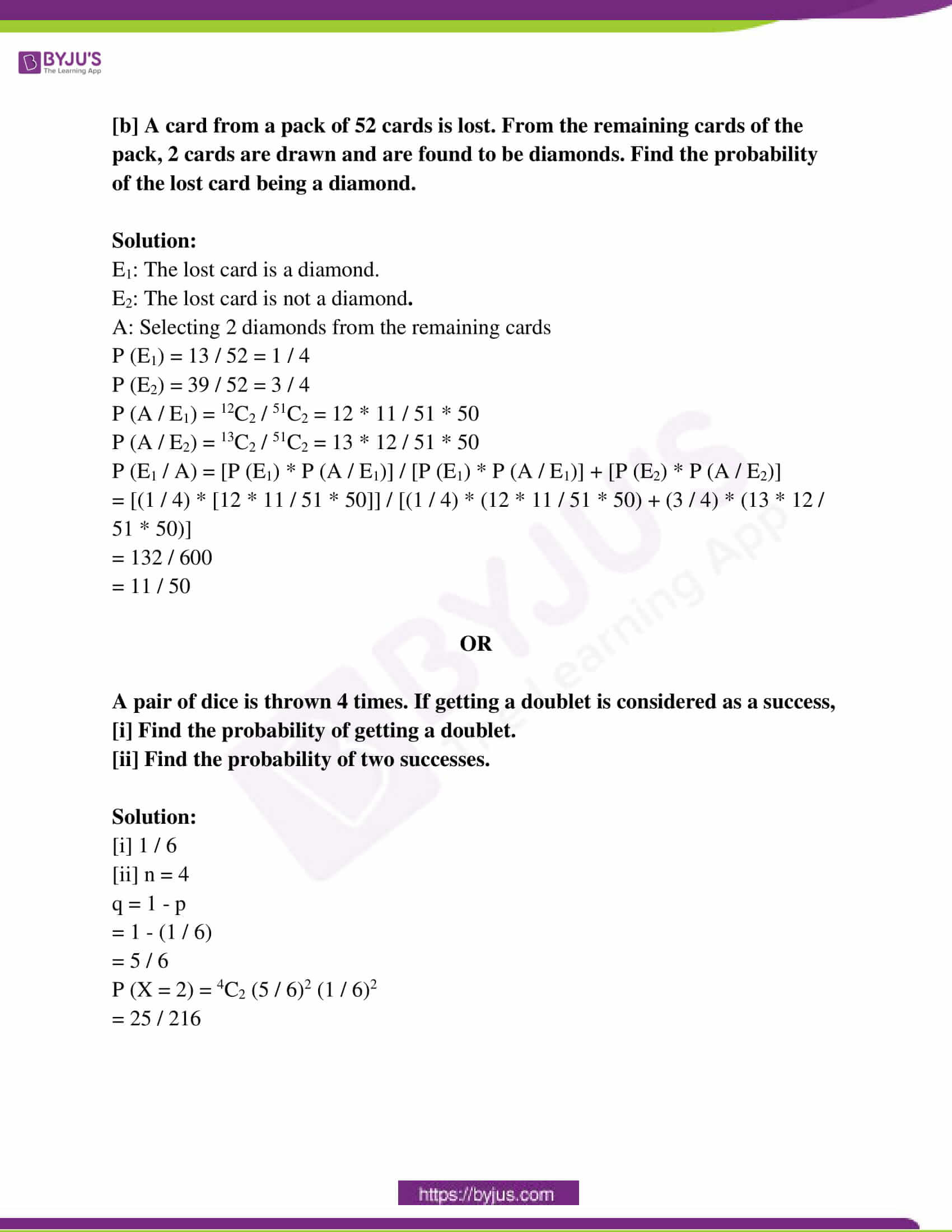Question 1[a]: The function f:N → N, given by f (x) = 2x is

(a) one-one but onto (b) one-one but not onto

(c) not one-one not onto (d) onto but not one-one

[b]: Find g o f (x), if f (x) = 8x3 and g (x) = x1/3.

Solution:

g o f (x) = g (f ( x))

= g (8x3)

= (8x3)

= 2x

[c]: Let * be an operation such that a * b = LCM of a and b defined on the set A = {1, 2, 3, 4, 5}. Is * a binary operation? Justify your answer.

Solution:

a * b = LCM of a and b

a, b ∈ {1, 2, 3, 4, 5}

Let a = 2, b = 3

a * b = 2 * 3 = LCM of 2 and 3 is 6

Since the element 6 doesn’t exist in the set {1, 2, 3, 4, 5}, * is not a binary operation.

Question 2[a]: If xy < 1, tan-1 x + tan-1 y is ______.

Solution:

tan-1 [(x + y) / (1 – xy)]

[b] Prove that 2 tan-1 (1 / 2) + tan-1 (1 / 7) = tan-1 (31 / 17)

Solution:

2 tan-1 (1 / 2) + tan-1 (1 / 7)

= tan-1 [(2 * (1 / 2)) / (1 – (1 / 2)2] + tan-1 (1 / 7)

= tan-1 (4 / 3) + tan-1 (1 / 7)

= tan-1 [((4 / 3) + (1 / 7)) / (1 – (4 / 3) (1 / 7)]

= tan-1 [(31 * 21) / (21 * 17)]

= tan-1 (31 / 17)

Question 3[a]: If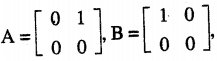then BA =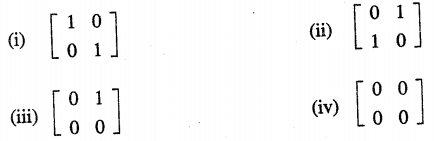Solution: [iii]

[b] Write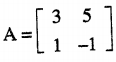as the sum of a symmetric and a skew-symmetric matrix.

Solution: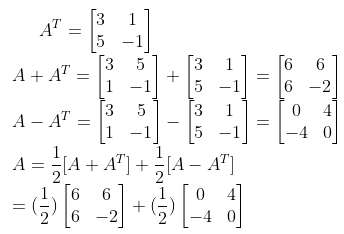[c] Find the inverse of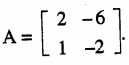Solution: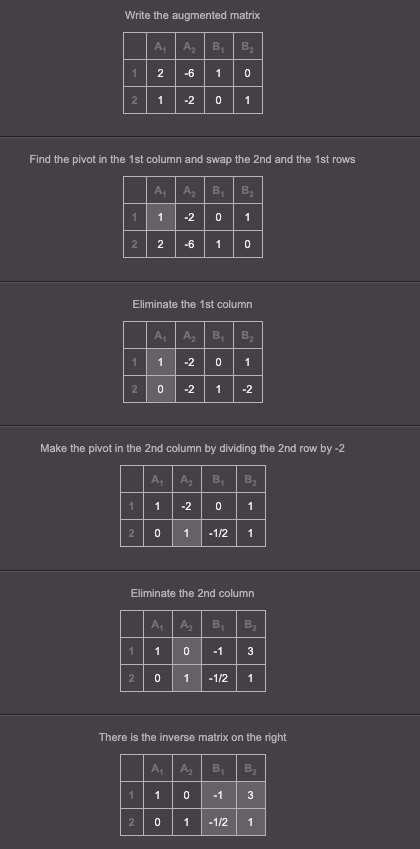Question 4[a]: The value of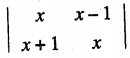is

(i) 1 (ii) x (iii) x2 (iv) 0

[b] Using the properties of determinants, show that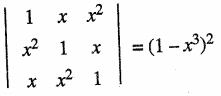.

Solution: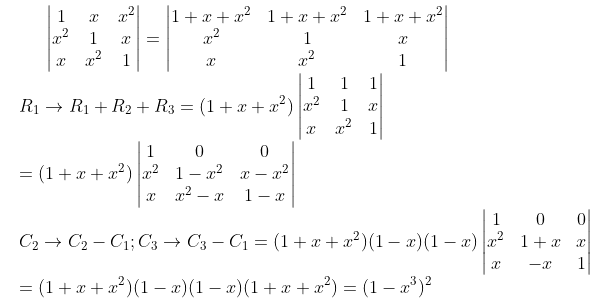Question 5[a]: Find all the points of discontinuity of f, where f is defined by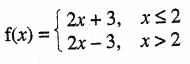Solution:

LHL = lim x→2- f (x) = lim x→2- 2x + 3 = 7

RHL = lim x→2+ f (x) = lim x→2+ 2x – 3 = 1

lim x→2- f (x) ≠ lim x→2+ f (x)

LHL ≠ RHL

2 is a point of discontinuity.

[b] If ex-y = xy, then prove that dy / dx = log x / (log ex)2.

Solution:

(x – y) log e = y log x

x – y = y log x

x = y log x + y

x = y (log x + 1)

y = x / (log x + 1)

dy / dx = (1 + log x – 1) / (1 + log x)2 = log x / (1 + log x)2

= log x / (log ex)2

Question 6[a]: The slope of the tangent to the curve given by x = 1 – cos θ, y = θ – sin θ at θ = 𝛑 / 2 is

(i) 0 (ii) -1 (iii) 1 (iv) Not defined

Solution: (ii)

[b] Find the intervals in which the function f (x) = x2 – 4x + 6 is strictly decreasing.

Solution:

f ‘ (x) = 2x – 4

f ‘ (x) = 0

2x – 4 = 0

x = 2

The intervals are (-∞, 2) and (2, ∞).

f (x) is decreasing at (-∞, 2).

[c] Find the minimum and maximum value, if any, of the function f (x) = (2x – 1)2 + 3.

Solution:

f (x) = (2x – 1)2 + 3

f ‘ (x) = 4 (2x – 1)

f ‘’ (x) = 8

f ‘ (x) = 0

2x – 1 = 0

x = 1 / 2

f (x) has minimum value at x = 1 / 2.

OR

[a] Which of the following functions has neither local maxima nor local minima?

(i) f (x) = x2 + x (ii) f (x) = log x

(iii) f (x) = x3 – 3x + 3 (iv) f (x) = 3 + |x|

Solution: [ii]

[b] Find the equation of the tangent to the curve y = 3x2 at (1, 1).

Solution:

y = 3x2

dy / dx = 6x

Slope at (1, 1) = 6

Equation of the tangent is y – y1 = (dy / dx)(1, 1) (x – x1)

y – y1 = 6 (x – 1)

y = 6x + 5

[c] Use differential to approximate √36.6.

Solution:

y = √x

x = 36

Δx = 0.6

dy = (dy / dx) Δx = 1 / 2 * √x

= 1 / 2 * (0.6)

= 0.05

√36.6 = 6 + 0.05 = 6.05

Question 7[a]: The angle between the vectors a and b such that |a| = |b| = √2 and a . b = 1 is

(i) 𝛑 / 2 (ii) 𝛑 / 3 (iii) 𝛑 / 4 (iv) 0

Solution: [ii]

[b] Find the vector along a – b where a = i + 3j – k and b = 3i + 2j + k.

Solution:

Unit vector along a – b = (a – b) / |a – b|

a – b = -2i + j – 2k

|a – b| = √9 = 3

Unit vector = (1 / 3) (-2i + j – 2k)

Question 8[a]: If the points A and B are (1, 2, -1) and (2, 1, -1) respectively, then AB is

(i) i + j (ii) i – j (iii) 2i + j – k (iv) i + j + k

Solution: [ii]

[b] Find the value of ƛ for which the vectors 2i – 4j + 5k, i – ƛj + k and 3i + 2j – 5k are coplanar.

Solution: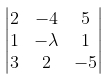= 0

2 (5ƛ – 2) + 4 (- 5 – 3) + 5 (2 + 3ƛ) = 0

ƛ = 26 / 25

[c] Find the angle between the vectors a = i + j – k and b = i – j + k.

Solution:

cos θ = (a . b) / |a| |b|

(a . b) = -1

|a| = √3

|b| = √3

cos θ = – 1 / 3

θ = cos-1 (- 1 / 3)

Question 9[a]: Prove that ∫cos2 x dx = (x / 2) + (sin 2x / 4) + c.

Solution:

cos2 x = (1 + cos 2x) / 2

∫cos2 x dx = (1 / 2) ∫(1 + cos 2x) dx

= (1 / 2) [x + (sin 2x / 2)] dx

[b] Find ∫dx / √2x – x2.

Solution:

2x – x2 = – (x2 – 2x)

= – (x2 – 2x + 1 – 1)

= – [(x – 1)2 – 12]

= 1 – (x – 1)2

∫dx / √2x – x2 = ∫dx / √1 – (x – 1)2

= sin-1 (x – 1) + c

[c] Find ∫x cos x dx.

Solution:

∫x cos x dx

= x sin x – ∫ sin x dx

= x sin x + cos x + c

Question 10[a]: Evaluate ∫0𝛑 log (1 + cos x) dx.

Solution:

I = ∫0𝛑 log (1 + cos x) dx

= ∫0𝛑 log (1 + cos [𝛑 – x] dx)

= ∫0𝛑 log (1 – cos x) dx

= ∫0𝛑 log 2 sin2 [x / 2] dx

= ∫0𝛑 (log 2 + 2 log sin (x / 2)) dx

= ∫0𝛑 log 2 dx + 2 ∫0𝛑 log sin (x / 2) dx

Put x / 2 = t

dx = 2 dt

When x = 0, t = 0 and x = 𝛑, t = 𝛑 / 2

I = log 2 (x)0𝛑 + 2 ∫0𝛑/2 log sin t * 2 dt

= log 2 (𝛑 – 0) + 4 (- 𝛑 / 2 log 2)

= – 𝛑 log 2

OR

[b] Find ∫05 (x + 1) dx as limit of sum.

Solution:

ab f (x) dx = lim h→0 h [f (a) + f (a + h) + ….. + f (a + (n – 1)h]

05 (x + 1) dx = lim h→0 h [1 + (1 + h) + (1 + 2h) + ….. 1 + (n – 1)h]

= lim h→0 h [n + h (1 + 2 + 3 ….. + (n – 1))

= lim h→0 h [n + h * n (n – 1) / 2]

= lim h→0 [nh + (n2h2 – h2n) / 2]

= lim h→0 [nh + nh (nh – h) / 2]

= lim h→0 (5 + [5 – h]5 / 2]

= 35 / 2

Question 11[a]: The area bounded by the curve y = f (x), above the x-axis, between x = a and x = b is

(i) ∫f(a)b y dy (ii) ∫af(b) x dx (iii) ∫ab x dy (iv) ∫ab y dx

Solution: [iv]

[b] Find the area of the circle x2 + y2 = 4 using integration.

Solution: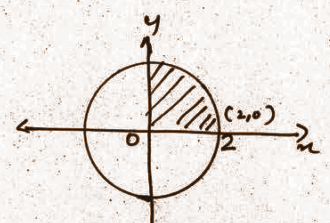Area of the shaded region = ∫02 y dx

= ∫02 √4 – x2 dx

= [(x / 2) √4 – x2 + (4 / 2) sin-1 (x / 2) dx

= (4 / 2) sin-1 (1)

= 2 * (𝛑 / 2)

= 4𝛑

Question 12[a]: y = cos x + b sin x is the solution of the differential equation.

(i) d2y / dx2 + y = 0 (ii) d2y / dx2 – y = 0

(iii) dy / dx + y = 0 (iv) dy / dx + x (dy / dx) = 0

Solution: [i]

[b] Find the solution of the differential equation x (dy / dx) + 2y = x2 (x ≠ 0) given that y = 0 when x = 1.

Solution:

(dy / dx) + (2y / x) = x

P = (2 / x), Q = x

IF = e∫P dx

= e(2 log x)

= x2

The solution is y = x2 = ∫x * x2 dx = ∫x3 dx

yx2 = x4 / 4 + c

When x = 1, y = 0,

0 = (1 / 4) + c

c = (- 1 / 4)

The particular solution is yx2 = (x4 / 4) + (- 1 / 4)

Question 13[a]: Find the shortest distance between the lines r = i + j + ƛ (2i – j + k) and r = 2i + j – k + 𝛍 (3i – 5j + 2k).

Solution:

Shortest distance = |[(a2 – a1) . (b1 – b2)] / |[b1 x b2]||

a1 = i + j

a2 = 2i + j – k

b1 = 2i – j + k

b2 = 3i – 5j + 2k

(a2 – a1) = i – k

[b1 x b2] = 3i – j + 7k

|[b1 x b2]| = √59

Shortest distance = 10 / √59

Question 14[a]: Equation of the plane with intercepts 2, 3, 4 on the x, y and z-axis respectively is

(i) 2x + 3y + 4z = 1 (ii) 2x + 3y + 4z = 12

(iii) 6x + 4y + 3z = 1 (iv) 6x + 4y + 3z = 12

Solution: [iv]

[b] Find the cartesian equation of the plane passing through the points A (2, 5, -3), B (- 2, – 3, 5) and C (5, 3, – 3).

Solution:

The equation is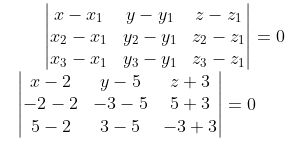(x – 2) 16 + (y – 5) 24 + (z + 3) 32 = 0

2x + 3y + 4z = 7

Question 15[a]: Consider the following LPP:

Maximise Z = 3x + 2y

Subject to constraints

x + 2y ≤ 10

3x + y ≤ 15

x, y ≥ 0

[a] Draw the feasible region.

[b] Find the corner points of the feasible region.

[c] Find the maximum value of Z.

Solution:

[a]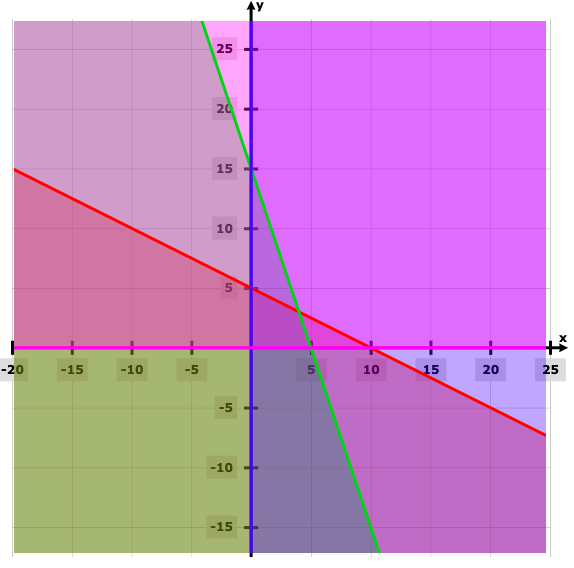[b] The corner points are (0, 0), (4, 3), (0, 5).

[c] Z is maximum at (4, 3) = 18

Question 16[a]: If P (A) = 0.3, P (B) = 0.4, then the value of P (A ⋃ B) where A and B are independent events is

(i) 0.48 (ii) 0.51 (iii) 0.52 (iv) 0.58

Solution: [iv]

[b] A card from a pack of 52 cards is lost. From the remaining cards of the pack, 2 cards are drawn and are found to be diamonds. Find the probability of the lost card being a diamond.

Solution:

E1: The lost card is a diamond.

E2: The lost card is not a diamond.

A: Selecting 2 diamonds from the remaining cards

P (E1) = 13 / 52 = 1 / 4

P (E2) = 39 / 52 = 3 / 4

P (A / E1) = 12C2 / 51C2 = 12 * 11 / 51 * 50

P (A / E2) = 13C2 / 51C2 = 13 * 12 / 51 * 50

P (E1 / A) = [P (E1) * P (A / E1)] / [P (E1) * P (A / E1)] + [P (E2) * P (A / E2)]

= [(1 / 4) * [12 * 11 / 51 * 50]] / [(1 / 4) * (12 * 11 / 51 * 50) + (3 / 4) * (13 * 12 / 51 * 50)]

= 132 / 600

= 11 / 50

OR

A pair of dice is thrown 4 times. If getting a doublet is considered as a success,

[i] Find the probability of getting a doublet.

[ii] Find the probability of two successes.

Solution:

[i] 1 / 6

[ii] n = 4

q = 1 – p

= 1 – (1 / 6)

= 5 / 6

P (X = 2) = 4C2 (5 / 6)2 (1 / 6)2

= 25 / 216